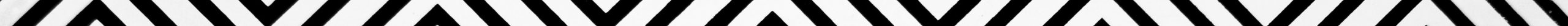Alternate Interior Angles

Area of a Trapezoid

Area of a Triangle

Area of Circle

Arithmetic Sequence

Circumference of Circle

Commutative Property

Complementary Angles

Compound Interest Formula

Decimal to Fraction

Exponential Form

Geometric Sequence

How to Multiply Fractions?

Interval Notation

Math Properties

Midpoint Formula

Multiplication Table

Orthocenter

Perimeter of a Rectangle

Point Slope Form

Slope Intercept Form

Supplementary Angles

Surface Area of a Cylinder

Trig Identities

X-Intercept

Y-InterceptInsert math as
$${}$$